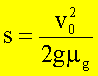# Why is friction important

## Experimental determination of the coefficient of static friction

friction

Internal friction or viscosity:

Viscosity is understood as the occurrence of tangential forces which counteract a displacement of particles in a liquid or a gas relative to one another.

External friction:

External friction is an interaction between bodies that occurs on the contact surface (or at the points of contact) and counteracts mutual displacement.

The subject of the following considerations is external friction.

Static friction:

Static friction occurs between bodies that are not moving relative to one another. If a body is to be moved relative to another, a swelling force must be used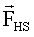are spent: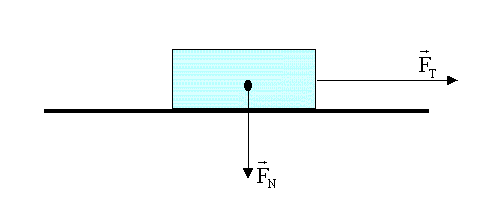Coulomb's law describes the relationship between

the swelling force, the normal force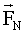and the coefficient of static friction µHas well as the acting tangential force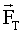: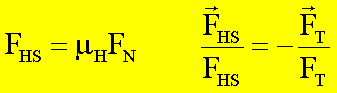·       If,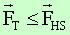according to the principle actio = reactio: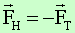·       If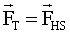, the static friction force is calculated according to Coulomb's law. This law is important insofar as it allows the maximum possible static friction force to be calculated.

·       If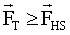so the body will move. In this case, other friction phenomena occur, e.g. sliding friction or rolling friction.

The amount of the maximum static friction force depends on the normal force(often, but not generally identical to the weight G) and on the properties of the contacting surfaces - expressed by µH. The coefficient of static friction is determined by the roughness of the surfaces, the interaction forces between the surface atoms (especially with very smooth surfaces) and the properties of the medium between the surfaces (e.g. air, water or oil).

The direction of the static friction force is opposite to the direction of the acting force: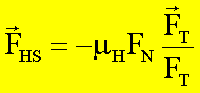The last formula contains both the amount and the direction of the maximum static friction force.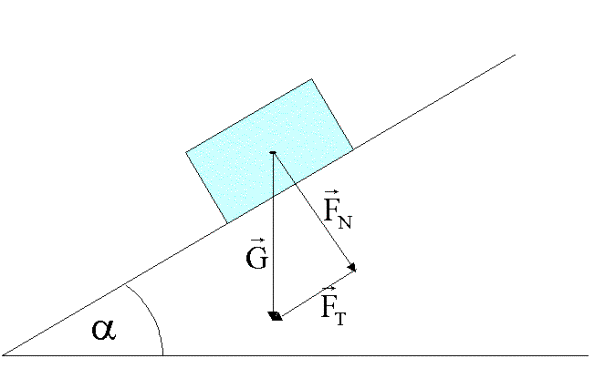In the experiment, the angle of inclination is increased until the downhill force (tangential force) is equal to the maximum static friction force. The following relationships apply: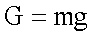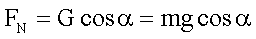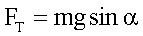If the body begins to slide, then the values ​​of the downhill force and the static friction force are exactly equal:, so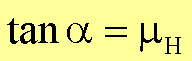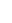Sliding friction:

### The sliding friction force acts when a body glides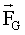. The sliding friction force is smaller than the static friction force: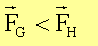A law analogous to static friction applies to dynamic friction:

A law analogous to static friction applies to dynamic friction: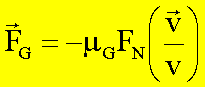The direction of the sliding friction force depends on the state of motion of the body. It is opposite to the current direction of speed, but does not depend on the amount of speed at low speeds. However, this law no longer applies to high speeds. The frictional force is then dependent on the amount of speed. The amount of the coefficient of sliding friction µG is smaller than the amount of the coefficient of static friction µH.

The coefficient of sliding friction can be determined experimentally, e.g. from the glide path s of a with the initial velocity v0 moving body can be determined:

Since the acceleration when the sliding friction acts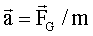is constant, the following relationships apply: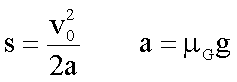This results in a braking distance of: